Get instant live expert help with Excel or Google Sheets“My Excelchat expert helped me in less than 20 minutes, saving me what would have been 5 hours of work!”

#### Post your problem and you’ll get Expert help in seconds.

Your message must be at least 40 characters
Our professional Expert are available now. Your privacy is guaranteed.

# Learn How to Add Time in Excel

Even the smartest people we know struggle with adding and subtracting time.  With Excel, this task has been made easy through the use of the TIME function.  Here’s how.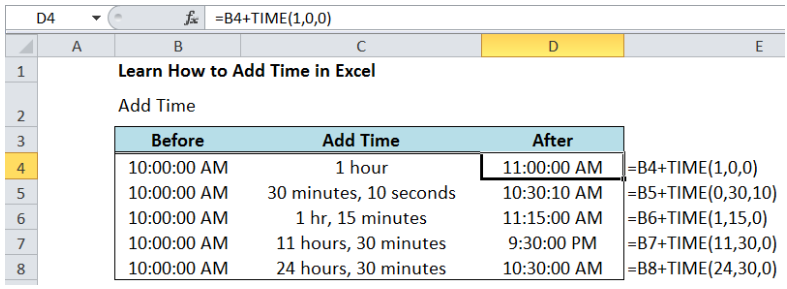Figure 1.  How to add time in Excel

## Setting up the Data

• Create a table with Before, Add Time and After as headers.  Enter the examples as shown in the table below. The Before time is 10:00:00 AM for all examples for us to have a simple and fixed reference.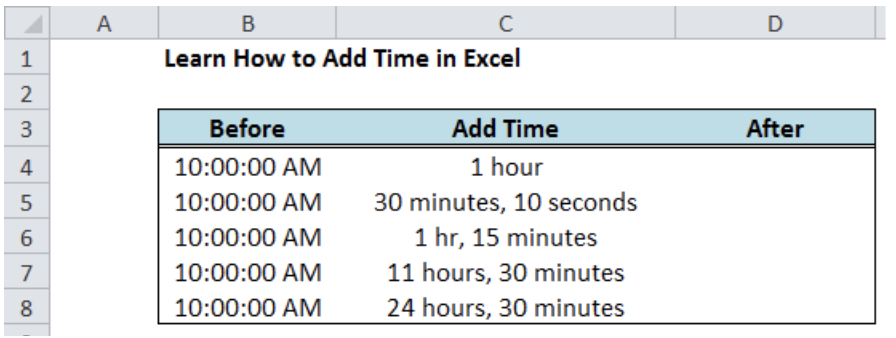Figure 2.  Sample Data for adding time in Excel

• Select cells B4:B8 and D4:D8
• Press Ctrl + 1 to show the Format Cells dialog box
• Select the Custom format h:mm:ss AM/PM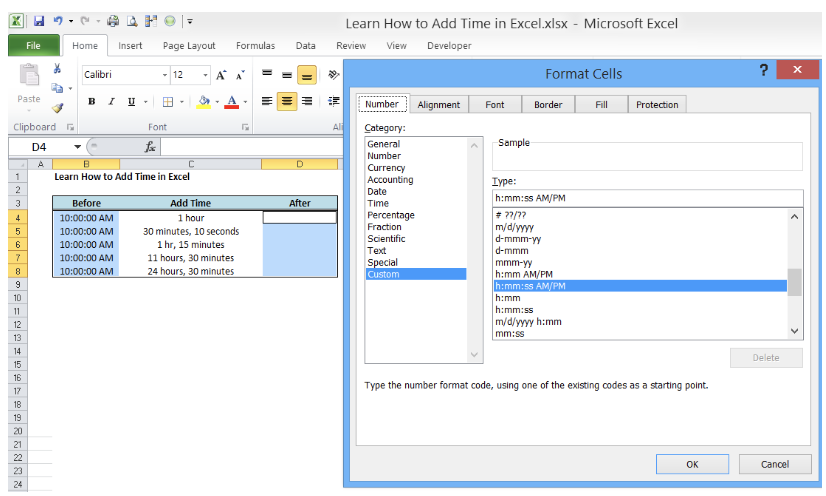Figure 3.  Setting the preferred time format

## What is TIME Function in Excel?

Time function is a built-in function in Excel that returns the decimal value of a particular time.  It is very useful when adding time because it allows compartmentalization of time into hours, minutes and seconds.

## Syntax

`=Time(hour, minute, second)`

When using this formula, we need to enter the time in this specific order : hours, minutes and then seconds.

## Add Time in Excel using the TIME Function

We want to add the time in column C to the “Before” time in Column B.

In cell D4, enter the formula:

`=B4+TIME(1,0,0)`

This formula adds 1 hour, 0 minutes and 0 seconds to 10:00:00 AM.  The result is 11:00:00 AM.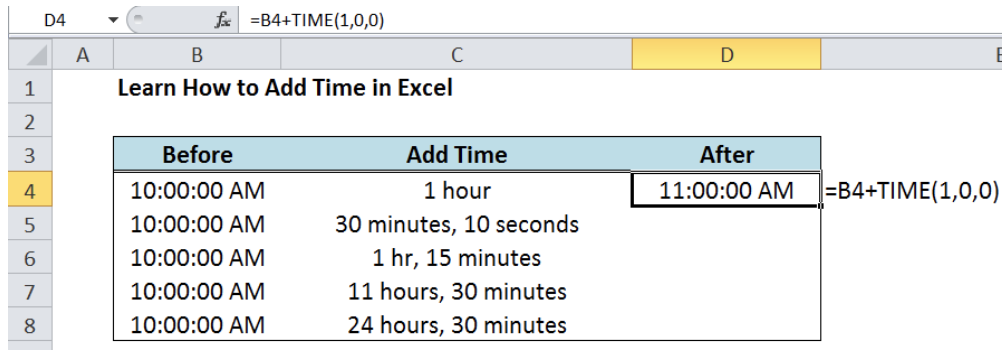Figure 4.  Entering the formula to add time in Excel

Through the TIME formula, it is very easy to add time in Excel.  Just follow the syntax and input the three arguments accordingly.  For the succeeding examples, add the TIME in hours, minutes and seconds as specified in column C.

The table below shows the corresponding formula and results.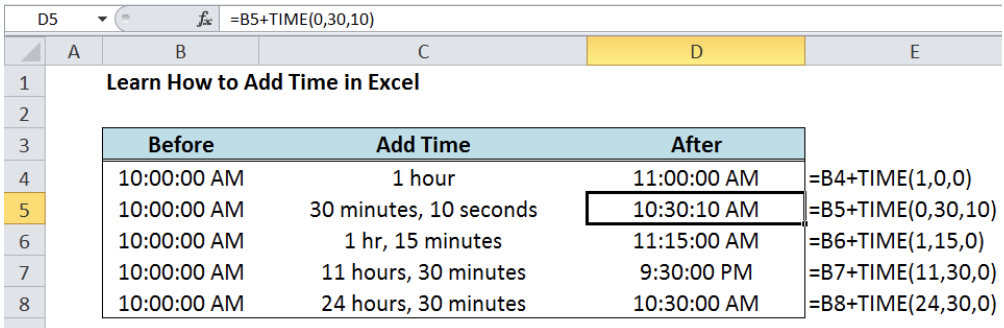Figure 5.  Output: Using the TIME function to add time in Excel

## Subtract Time in Excel using the TIME Function

Subtracting time is similar to adding time; the only difference is that the mathematical operation used is Subtraction and not Addition.

For the purpose of comparison, let us reverse the process and use the After data in the previous example as the Before data in this exercise.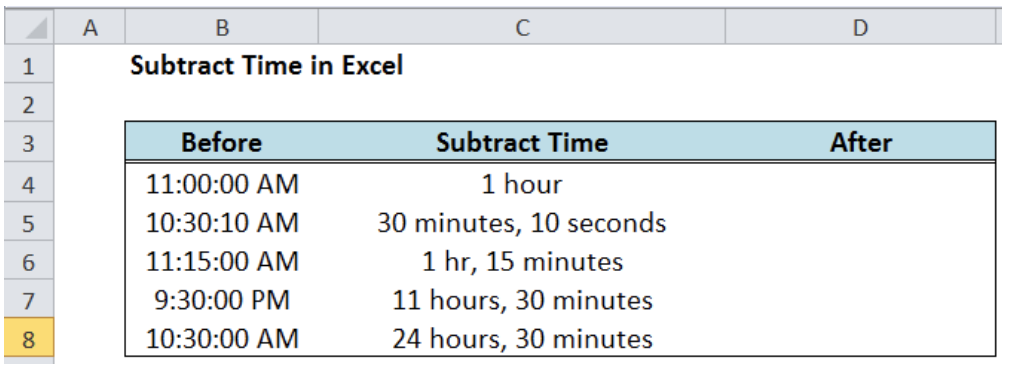Figure 6.  Sample data for subtracting time in Excel

We want to subtract the time in column C to the “Before” time in Column B.

In cell D4, enter the formula:

`=B4-TIME(1,0,0)`

This formula subtracts 1 hour, 0 minutes and 0 seconds to 11:00:00 AM.  The result is 10:00:00 AM.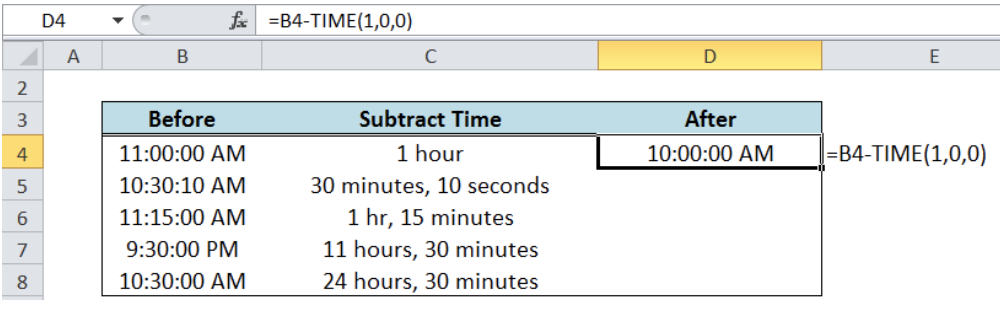Figure 7.  Entering the formula to subtract time in Excel

Do the same for the other examples to obtain below results: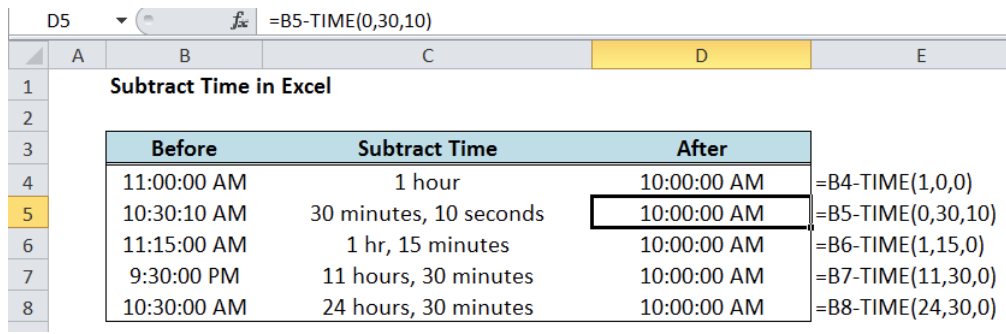Figure 8.  Output: Using the TIME function to subtract time in Excel

As shown above, we have successfully subtracted time and obtained the “After” time of 10:00:00 AM for all examples.

Most of the time, the problem you will need to solve will be more complex than a simple application of a formula or function. If you want to save hours of research and frustration, try our live Excelchat service! Our Excel Experts are available 24/7 to answer any Excel question you may have. We guarantee a connection within 30 seconds and a customized solution within 20 minutes.

### Did this post not answer your question? Get a solution from connecting with the expert.Another blog reader asked this question today on Excelchat:
Solution examplesHello I have a list of codes, some of these a duplicated in one column. In another column I have a list of prices. I am trying to work out how I identify when the code and two prices match and when they are different please? thank you
Solved by O. C. in 40 minsI need to calculate the number of female board members and directors for each company, also the % of females and how they compare to number and % of men in the same firms. Only small firms should count and only board members and relevant associating functions.
Solved by K. D. in 41 minsI need to create a sheet like Column one # Column 2 Month Column 3 Date Column 4 Amount Column 5 Amount Column 6 is total of #4 n #5 Column 7 amount Column 8 is #5 reduce from # 7 any one can help me please
Solved by S. W. in 11 minsI'd like to create a set of random numbers that are selected based on an assigned probability. For example, if a biologist wants to visit two ends of a lake, one 80 percent of the time and the other 20 percent of the time but on random days,how do I create this schedule?
Solved by O. D. in 29 minscopying numbers from 1 column to another in filter mode brings irrelevant data over to new column
Solved by O. J. in 40 mins## Subscribe to Excelchat.coAnother blog reader asked this question today on Excelchat: